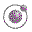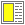stream-stuff.scm - All Scheme stream stuff collected in one file. Lecture 5 - slide 24 : 26Program 2

```(define-syntax cons-stream
(syntax-rules ()
((cons-stream x y)
(cons x (delay y)))))

(define (tail stream) (force (cdr stream)))

(define empty-stream? null?)

(define the-empty-stream '())

(define (stream-section n stream)
(cond ((= n 0) '())
(else (cons (head stream)
(stream-section
(- n 1)
(tail stream))))))

(define (add-streams s1 s2)
(let ((h1 (head s1))